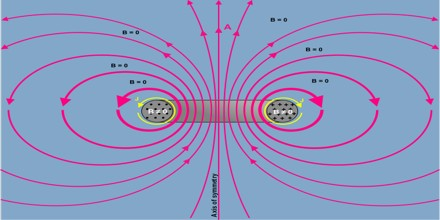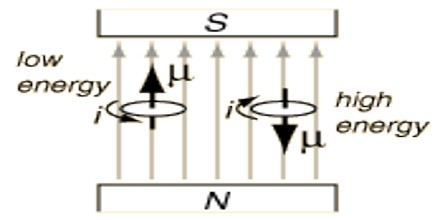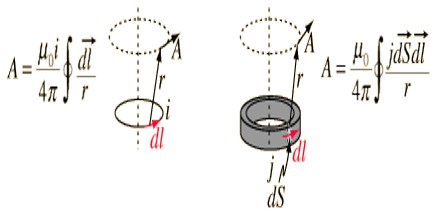Magnetic Potential

Definition

Magnetic Potential is the property of certain substances or phenomena that gives rise to magnetic fields. Magnetic Potential, also known as Magnetomotive Force, which is analogous to electromotive force or voltage in electricity. The magnetic vector potential, A, (often simply called the vector potential) and the magnetic scalar potential, ψ. Both quantities can be used in certain circumstances to calculate the magnetic field.The standard definition of magnetomotive force (or magnetic potential) involves current passing through an electrical conductor, permanent magnet s also exhibit magnetomotive force. The same is true for planets with magnetic fields, such as the Earth, Jupiter, Saturn, Uranus, and Neptune. The Sun also generates magnetomotive forces, particularly in the vicinity of sunspots.

The standard unit of magnetomotive force is the Ampere-Turn (AT), represented by a steady, direct electrical current of One Ampere (1 A) flowing in a single-turn loop of electrically conducting material in a vacuum. Sometimes a unit called the Gilbert (G) is used to quantify magnetomotive force. The gilbert is defined differently, and is a slightly smaller unit than the ampere-turn. To convert from ampere-turns to gilberts, multiply by 1.25664. Conversely, multiply by 0.795773.Energy of Magnetic Potential

A magnetic dipole in a magnetic field has potential energy given by

U=−μB

The dipole will feel a torque, τ = μ×B, that will tend to align it with the field so as to minimize U. If the magnetic field is not uniform, it will also feel a force equal to the negative gradient of the potential energy, (μB).

This is the lowest order term in a multipole expansion: there is also a quadrupole term − 1/6 Qij∂iBj and so on.

The energy is expressed as a scalar product, and implies that the energy is lowest when the magnetic moment is aligned with the magnetic field.

When ever a particle or an object come in the influence of some field or particle then it interacts with the field or particle. for example if a charge come near another charge then it feels electrostatic potential similarly if a charge particle is placed in magnetic field it interacts with magnetic field this interaction energy is magnetic potential energy.Magnetic Vector and Scalar Potential

The physical meaning of the magnetic vector potential is actually very similar: it’s the potential energy per unit element of current. By “element of current” what is meant is a quantity with the units of current times length; it’s the current through a piece of wire times the length of the wire, with the direction of conventional current. Accordingly, the units of the magnetic vector potential are joules per ampere-meter in SI.

The electric potential energy is well-known: it’s given by the formula

Ue = ½ ρϕdV

This formula justifies the idea that the electric scalar potential is energy per unit charge. ρdV is an infinitesimal element of charge, multiplying it by ϕ gives the energy.

It also shows that the energy required to assemble a charge distribution doesn’t depend on how the charges got there (provided there’s no friction).

There’s also magnetic potential energy. It takes energy to fire up a current in a wire because of the wire’s self-inductance: increasing the current induces a back emf and you have to pump energy into the system in order to overcome it. It also takes energy to reposition two current-carrying wires relative to each other as they exert magnetic forces on each other.

The magnetic vector potential also has a deeper meaning, and it’s the phase shift per unit charge that a charged particle picks up as it moves through space. That is, if a particle with charge q moves along a path from one point to another, the magnetic vector potential causes the phase of its quantum-mechanical wave function to change by